Search IntMath
Close

450+ Math Lessons written by Math Professors and Teachers

5 Million+ Students Helped Each Year

1200+ Articles Written by Math Educators and Enthusiasts

Simplifying and Teaching Math for Over 23 Years

# Volume of a pendant

By Murray Bourne, 19 Nov 2012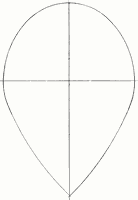Reader JT wrote with an interesting problem recently.

He has a 3-dimensional pendant with the cross-sectional shape shown, and he wanted to know its volume.

This requires knowledge of Volume of Solid of Revolution, which uses calculus.

The basic idea is we take the given shape and rotate it around the y-axis. This is one way to actually manufacture such a pendant - by putting a block of material on a lathe and spinning it, shaving off the edges until we achieve the desired 3-dimensional object.

Mathematically, we actually slice the 3-D object horizontally into many (in fact, infinite) super-slim cylinders. We add the volumes of those thin slices to get the volume of the pendant. To do this, we need to know the function expressions for each portion of the pendant and apply integration.

JT supplied a diagram which showed where the cross-sectional shape came from. It's derived from 3 circles, as follows:

The top portion, M to K, is an arc of the (black) circle center F (0,2) and radius 5.17 cm.

The middle portion, K to H, is an arc of the (light blue) circle center G (-2,0) with radius 8 cm.

The bottom portion, H to J, is an arc of the red circle with center A (not shown) at (-18,7.75) and radius 25.78 cm.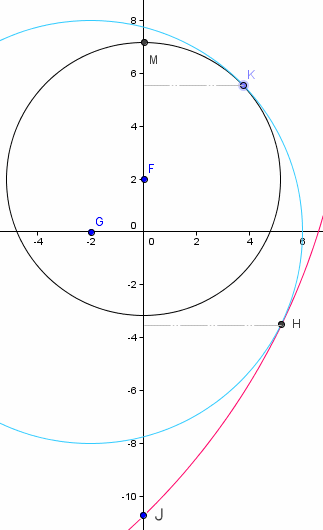(I used GeoGebra to create the above diagram and the other graphs in this article. It's a very useful tool for this kind of problem.)

Here's the final result after merging the arcs and reflecting the result in the y-axis.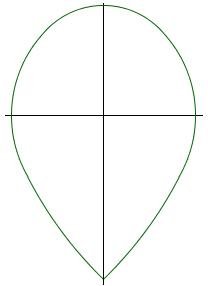Now for the volume.

## Volume approximation

In these problems, it's always a good idea to make an estimate of the answer so we can check our work later.

When we rotate the above shape around the y-axis, we see its shape will be close to a sphere with radius about 6.5 cm. In general, the volume of a sphere is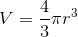Substituting r = 6.5 gives us 1150.3 cm3.

## Volume of Solid of Revolution - vertical axis

Here is the formula we use.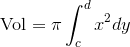It involves expressing the function in terms of x (so it will have terms in the variable y only), squaring, and then integrating.

Since the edge of the pendant is in 3 pieces, we need to break up the integral into 3 bits (I'm working bottom to top and using the y-values of the arc intersection points for the upper and lower bounds in each integral):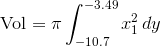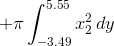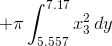Now for the functions.

Our aim is to find the expression for x2 for the 3 different arcs.

In general, the circle with center (h,k) and radius r has the equation: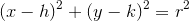I'll use the simplest case (the middle arc) to illustrate what we need to do. In our example, the middle arc is part of a circle with center G (-2,0) and radius 8. It has equation: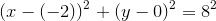That is: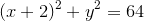Some basic algebra gives us the expression for x2 (which is in terms of y):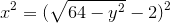(I'm taking the positive square root only since we just need the right hand portion of the pendant.) I'll use this for x2 in my volume integral.

Applying similar steps for the other 2 arcs, and substituting those results in the volume equation gives us: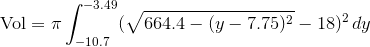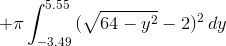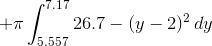Next, we use a computer algebra system (I used Scientific Notebook, or you could use Wolfram|Alpha) to evaluate this integral and we find the volume to be 1140.76 cm3.

This is fairly close to our earlier estimate (of 1150) so we can be confident it is correct.

## Alternative Solution - Ellipse model

Another way you could do this is to draw an ellipse through the known points and use that as a good approximation to the circles. I expected it would be less work and still give a good solution.

The points M, K, H and J are known, so we can use GeoGebra to draw an ellipse through the points, like this.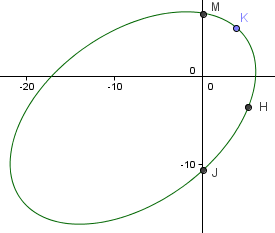Taking the portion to the right of the y-axis and spinning it around that axis gives the following cross-section. You can see it is close to the original pendant shape. The top is a bit pointed, but it won't make much difference to the volume.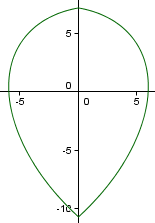GeoGebra tells us the formula of the ellipse:

-594.9x2 + 594.7xy - 800.5y2 - 6672.3x - 2828.1y + 61451.0 = 0

We'll need to solve this ellipse equation for x2 (I used Scientific Notebook to do this for me, by first solving for x and then squaring it):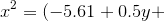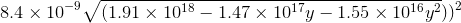So all we need to do now is substitute this expression into the volume formula. The lower and upper limits of the integral are -10.7 (point J) and 7.17 (point M).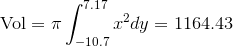So this method of using an ellipse to model the pendant gives quite a good estimate (only 2% too big). While it may look a bit messy here, it was actually less work overall.

### 2 Comments on “Volume of a pendant”

1. Pete Owen says:

Some pendants are solids of revolution, but many are of the given shape but flattened front and back. In such cases we might be forced to use Archimedes' water displacement technique. What do you think?

2. Murray says:

@Pete: Yes, I agree!

### Comment Preview

HTML: You can use simple tags like <b>, <a href="...">, etc.

To enter math, you can can either:

1. Use simple calculator-like input in the following format (surround your math in backticks, or qq on tablet or phone):
a^2 = sqrt(b^2 + c^2)
(See more on ASCIIMath syntax); or
2. Use simple LaTeX in the following format. Surround your math with $$ and $$.
$$\int g dx = \sqrt{\frac{a}{b}}$$
(This is standard simple LaTeX.)

NOTE: You can mix both types of math entry in your comment.